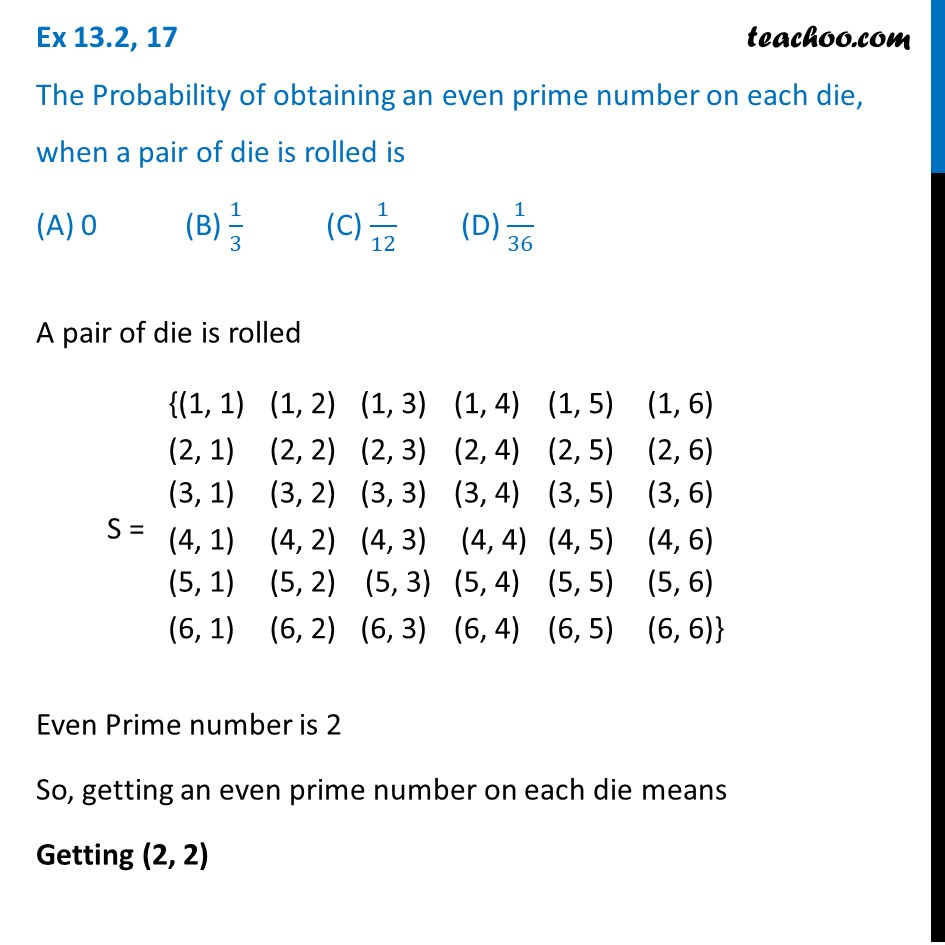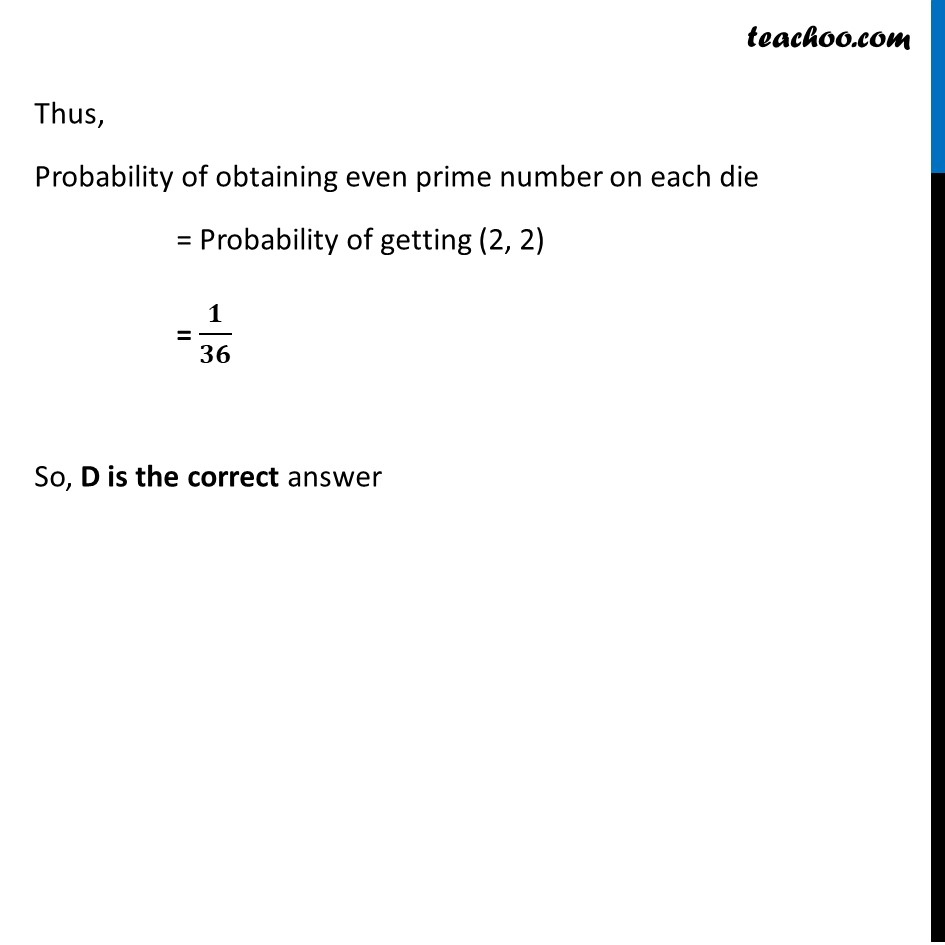Basic Probability

Chapter 13 Class 12 Probability
Concept wiseLearn in your speed, with individual attention - Teachoo Maths 1-on-1 Class

### Transcript

Ex 13.2, 17 The Probability of obtaining an even prime number on each die, when a pair of die is rolled is (A) 0 (B) 1/3 (C) 1/12 (D) 1/36 A pair of die is rolled S = Even Prime number is 2 So, getting an even prime number on each die means Getting (2, 2) Thus, Probability of obtaining even prime number on each die = Probability of getting (2, 2) = 𝟏/𝟑𝟔 So, D is the correct answer